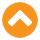# Power of Compounding - Compound Interest Calculator

Step 1

### I want to invest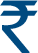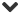Step 2

5 yrs 30 yrs
Step 3

10 yrs 30 yrs
Step 4

### I expect rate of return of (Annually)

1% 16%
after 15 Years @ 10% p.a.
IN UNIT LINKED INSURANCE PLANS, THE INVESTMENTS RISK IN THE INVESTMENT PORTFOLIO IS BORNE BY THE POLICYHOLDER
Pay 4,000 p.m. for 15 Years & get
Maturity Benefit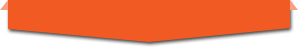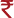13.5 lakh @ 8% assumed rate of return
OR10.8 lakh @ 4% assumed rate of return
Life Cover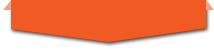15 lakh

#### How often is interest compounded?

The frequency of compounding may vary across banks. They usually calculate according to their own will. However, in practice, only a few methods of compounding are used:
Annual compounding: Interest is calculated once a year
Half-yearly compounding: Interest is calculated every six months
Quarterly compounding: Interest is calculated once every three months
Daily compounding: Interest is calculated every day

#### What does compounding interest mean?

Compounding interest means interest on interest. Each time you earn interest on your principal, it is added to the original amount, which then becomes the principal for the next cycle. This allows exponential growth for your interest. Compounding interest can be good or bad depending on whether you are a saver or a borrower, respectively.

#### Is it better to compound daily or monthly?

Although the difference won’t be much, it is better to opt for daily compounding. The interest amount in case of daily compounding will be slightly higher than the other options. This is governed by the principle: the shorter the compounding interval, the higher the interest.

#### How does compounding power work?

The power of compounding works by growing your wealth exponentially. It adds the profit earned back to the principal amount and then reinvests the entire sum to accelerate the profit earning process.
Suppose, you invest ₹ 1000 in a bank which offers 10% interest per annum. Your investment becomes ₹ 1100 after the first year, then ₹ 1210 after 2nd year and so on.

#### How often is interest compounded?

The frequency of compounding may vary across banks. They usually calculate according to their own will. However, in practice, only a few methods of compounding are used:
Annual compounding: Interest is calculated once a year
Half-yearly compounding: Interest is calculated every six months
Quarterly compounding: Interest is calculated once every three months
Daily compounding: Interest is calculated every day

#### What does compounding interest mean?

Compounding interest means interest on interest. Each time you earn interest on your principal, it is added to the original amount, which then becomes the principal for the next cycle. This allows exponential growth for your interest. Compounding interest can be good or bad depending on whether you are a saver or a borrower, respectively.

#### Is it better to compound daily or monthly?

Although the difference won’t be much, it is better to opt for daily compounding. The interest amount in case of daily compounding will be slightly higher than the other options. This is governed by the principle: the shorter the compounding interval, the higher the interest.

#### How does compounding power work?

The power of compounding works by growing your wealth exponentially. It adds the profit earned back to the principal amount and then reinvests the entire sum to accelerate the profit earning process.
Suppose, you invest ₹ 1000 in a bank which offers 10% interest per annum. Your investment becomes ₹ 1100 after the first year, then ₹ 1210 after 2nd year and so on.